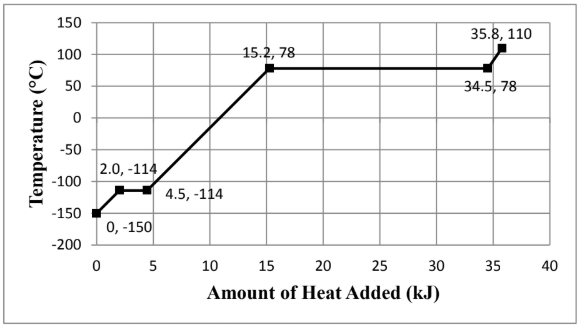# Problem: Consider the heating curve shown below for 22.86 grams of an unknown substance. You may assume constant pressure conditions. Note: the points are labeled as (amount of heat added, temperature). Calculate the amount of heat required (in kJ) to vaporize the compound whose heating curve is shown above. Show all work and circle your final answer.

###### FREE Expert Solution

We are asked to calculate the amount of heat to vaporize 22.86 grams of an unknown substance given the heating curve.

Phase changes occur on the horizontal parts of the curve since there are no temperature changes.

97% (364 ratings)###### Problem Details

Consider the heating curve shown below for 22.86 grams of an unknown substance. You may assume constant pressure conditions. Note: the points are labeled as (amount of heat added, temperature).Calculate the amount of heat required (in kJ) to vaporize the compound whose heating curve is shown above. Show all work and circle your final answer.

Frequently Asked Questions

What scientific concept do you need to know in order to solve this problem?

Our tutors have indicated that to solve this problem you will need to apply the Heating and Cooling Curves concept. You can view video lessons to learn Heating and Cooling Curves. Or if you need more Heating and Cooling Curves practice, you can also practice Heating and Cooling Curves practice problems.